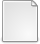# Improved Inverse Scaling and Squaring Algorithms for the Matrix Logarithm

Al-Mohy, Awad H. and Higham, Nicholas J. (2011) Improved Inverse Scaling and Squaring Algorithms for the Matrix Logarithm. [MIMS Preprint]There is a more recent version of this item available.PDF paper13.pdf Download (390kB)Other logm.zip Download (6kB)

## Abstract

A popular method for computing the matrix logarithm is the inverse scaling and squaring method, which essentially carries out the steps of the scaling and squaring method for the matrix exponential in reverse order. Here we make several improvements to the method, putting its development on a par with our recent version [\emph{SIAM J. Matrix Anal.\ Appl.}, 31 (2009), pp.\ 970--989] of the scaling and squaring method for the exponential. In particular, we introduce backward error analysis to replace the previous forward error analysis; obtain backward error bounds in terms of the quantities $\|A^p\|^{1/p}$, for several small integer $p$, instead of $\|A\|$; and use special techniques to compute the argument of the Pad\'e approximant more accurately. We derive one algorithm that employs a Schur decomposition, and thereby works with triangular matrices, and another that requires only matrix multiplications and the solution of multiple right-hand side linear systems. Numerical experiments show the new algorithms to be generally faster and more accurate than their existing counterparts and suggest that the Schur-based method is the method of choice for computing the matrix logarithm.

Item Type: MIMS Preprint To appear in SIAM J. Sci. Comp. matrix logarithm, inverse scaling and squaring method, matrix exponential, backward error analysis, Pad\'e approximation, matrix square root, MATLAB, \texttt{logm} MSC 2010, the AMS's Mathematics Subject Classification > 15 Linear and multilinear algebra; matrix theoryMSC 2010, the AMS's Mathematics Subject Classification > 65 Numerical analysis Nick Higham 20 Apr 2012 08 Nov 2017 18:18 http://eprints.maths.manchester.ac.uk/id/eprint/1799

### Available Versions of this ItemView Item